# Put workspace in docx

## Workshop documents

Documents designed for workshop or classroom participation typically provide information, examples, and prompts with room for participants to respond. The functions described here provide space in a document for written responses.

• For a prompt that requires a written response, the put_gap() function inserts a blank space in the document.
• For a prompt that requires a sketch in a coordinate frame, the put_axes() function inserts an empty coordinate frame in the output document.

Both functions work by creating and returning a ggplot2 object.

library("docxtools")

## put_gap()

This function allows the author of Rmd to docx documents to insert a vertical white space of specified height.

The white space is an empty ggplot2 object. The only assignment is the panel background, made with theme(panel.background = element_rect()).

The usage is put_gap(col = NULL, fill = NULL) to specify the color of the rectangle border and its fill. Defaults are “transparent”.

The dimensions of the gap are determined when it is printed. For example, to create a 0.75 inch gap in an R Markdown output document, we set the dimension in the knitr code chunk header,

{r fig.height = 0.75}
put_gap()


produces this output: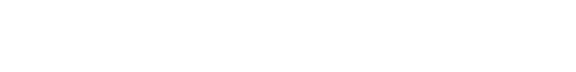With color and fill arguments and an optional width argument in the code chunk header,

{r fig.height = 0.75, fig.width = 6}
put_gap(col = "black", fill = "gray")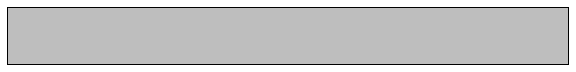Here’s an image from a Word document where space for written responses was created using put_gap().

knitr::include_graphics("../man/figures/example-docx.png")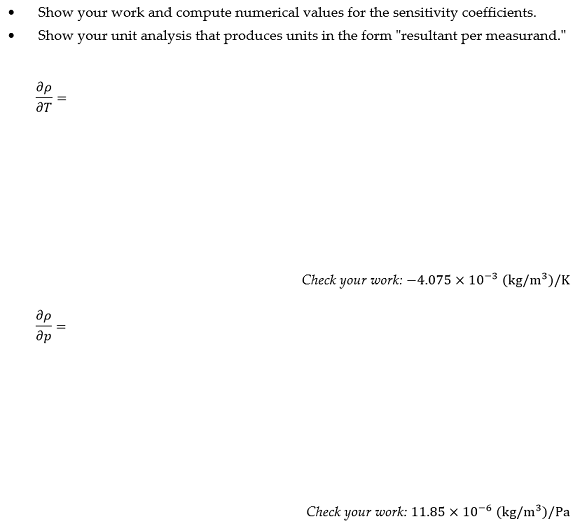A gap can also be used to force a page break. Alternatively, Norbert Köhler shows how to insert page breaks using Word Styles, see R Markdown: How to insert page breaks in a MS Word document.

## put_axes()

put_axes() creates and prints an empty, 2-dimensional coordinate axes using ggplot2 with no scales, no tick marks, and no axis labels.

Usage is put_axes(quadrant = NULL, col = NULL, size = NULL).

• quadrant 0 for all quadrants; 1, 2, 3, 4 for a single quadrant; or any combination of adjacent quadrants, e.g., 12, 21, 23, 34, etc. The default is 1.
• col is line color. Default is gray60.
• size is line size. Default is 0.5.
• the hidden scales have magnitude 1.

# all 4 quadrants
put_axes(0)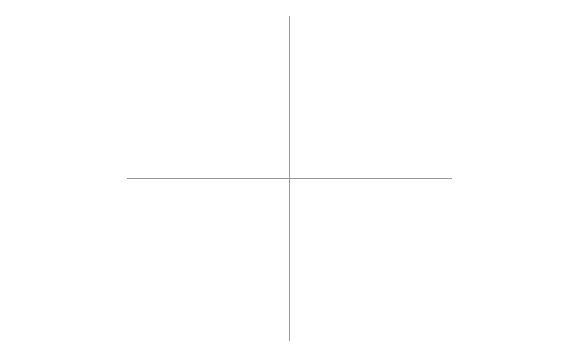# quadrant 2
put_axes(2)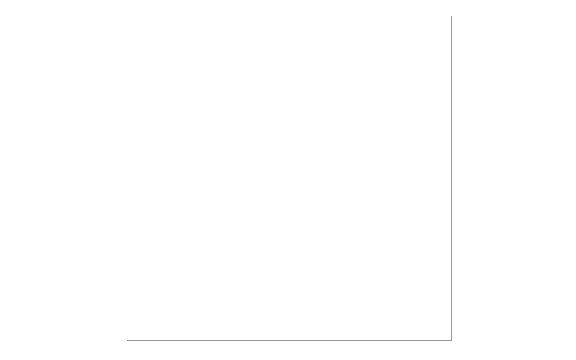# quadrant 1
put_axes(1, col = "blue", size = 2)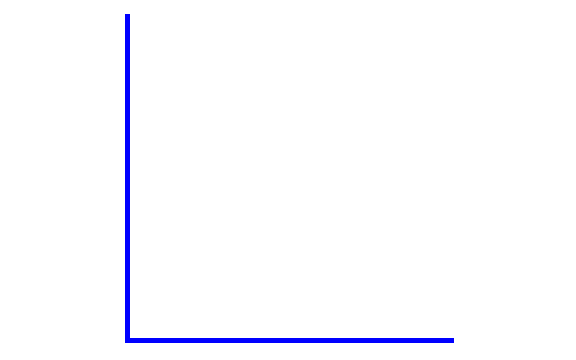# quadrant 3
put_axes(3)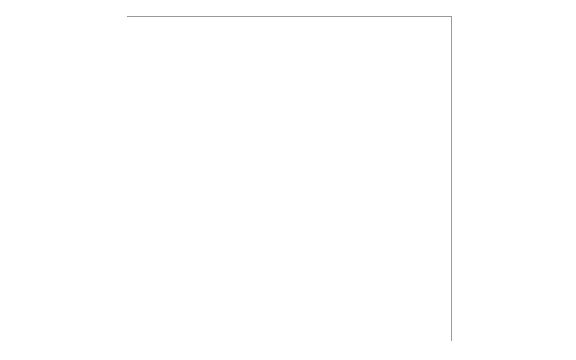# quadrant 4
put_axes(4, col = "red", size = 2)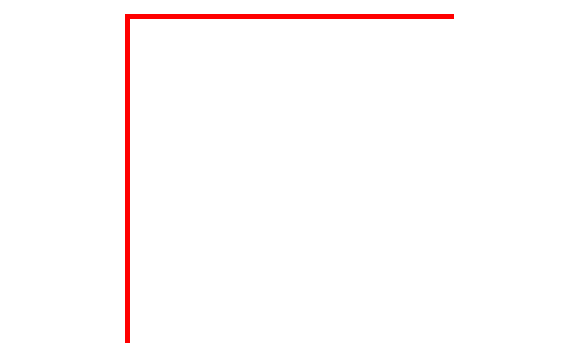# two adjacent quadrants
put_axes(12)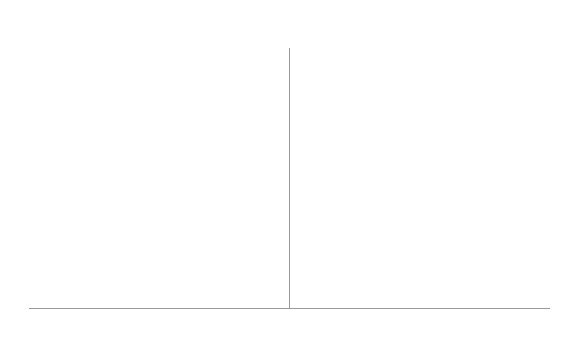# two adjacent quadrants
put_axes(41)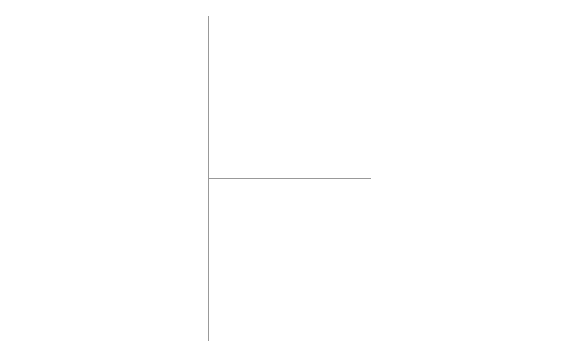### Dimensions

The size of the figure is determined when printed, e.g., using knitr in an R Markdown script, the figure height in inches is set with the fig.height code chunk option.

{r fig.height = 2}
put_axes()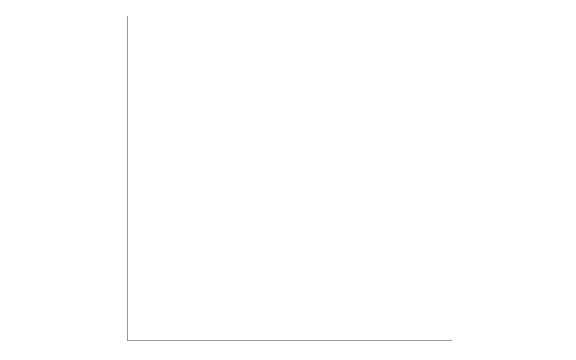The default aspect ratio is 1, though it can be edited using coord_fixed() from ggplot2.

library(ggplot2)
put_axes() +
coord_fixed(1 / 2) # ratio of y/x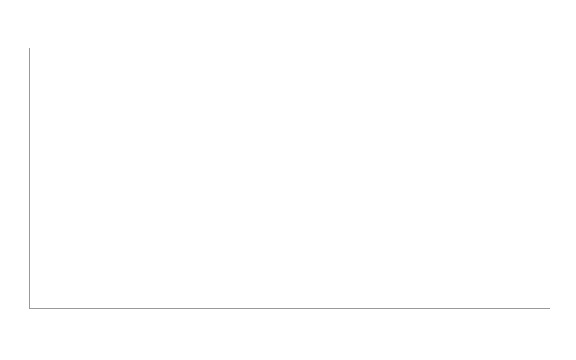### Annotations

The graph object can be edited using the usual ggplot2 annotations. For example, to place some text in the middle of the graph,

p <- put_axes() +
annotate("text", x = 0.5, y = 0.5, label = "quadrant I")
p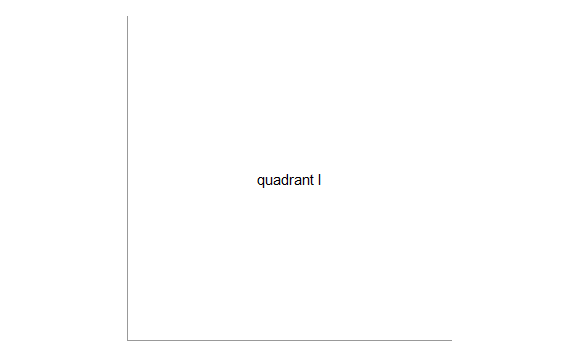We can add text labels to label the axes using annotate()

p <- put_axes() +
annotate("text",
x = c(1, 0), y =
c(0, 1), label =
c("x", "y"), vjust =
c(1, 0.5), hjust =
c(1, 1.5), fontface = "italic"
)
p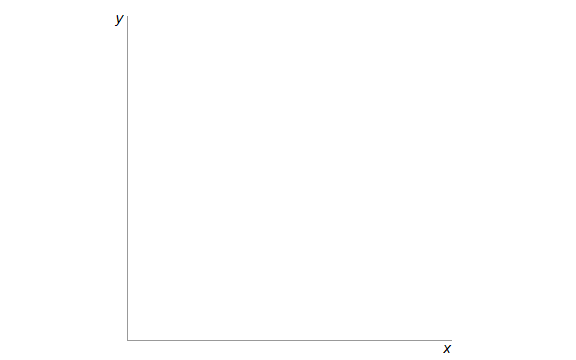Or we can use the usual labs() after resetting the theme axis.title,

p <- put_axes() +
theme(axis.title = element_text()) +
labs(x = "new x", y = "new y")
p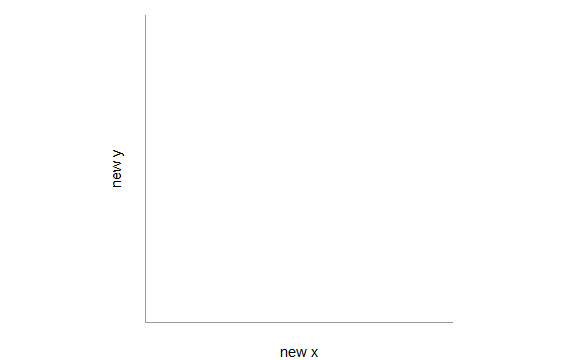If we use annotate() and the label exceeds the data rectangle, we can make the plot margin larger and override the plot clipping.

p <- put_axes(2) +
annotate("text",
x = c(-1, 0), y =
c(0, 1), label =
c("Re", "Im"), vjust =
c(1.5, 0.5), hjust =
c(0, -0.5), fontface = "italic"
) + # top, right, bottom, left
theme(plot.margin = unit(c(0, 5, 2, 0), "mm"))

# override clipping
library(grid)
pp <- ggplot_gtable(ggplot_build(p))
pp$layout$clip[pp$layout$name == "panel"] <- "off"
grid.draw(pp)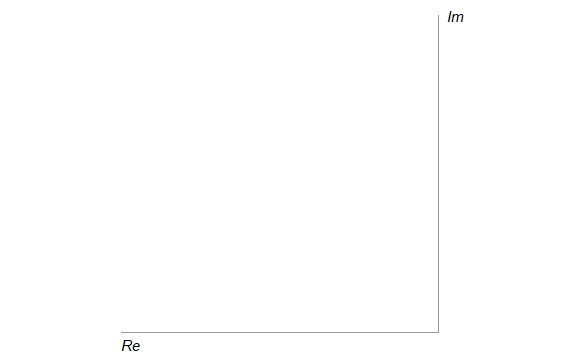## Conclusion

These functions help create printed documents with space for written responses. Options are limited; authors needing more control can create their own inserts using ggplot2 or other graphics engine.